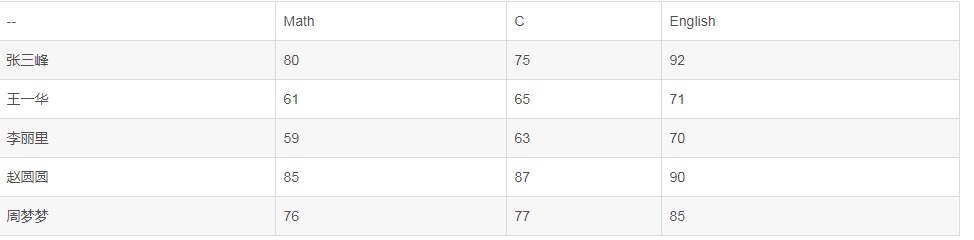• ②使用二重循环，对已经存在于二维数组 A 中的进行平均分计 算，将结果保存到一维 数组 B 中。 ③使用循环输出一维数组 B（即平均分）的java 数学 英语 体育 政治 001 95 89 79 64 76 002 80 88...java 二维数组 编程语言
• Java之二维数组求平均值

千次阅读 2017-12-03 22:12:05
public class test11{ public static void main(String[] args){ int[][] scores ={{79,98,88},{87,96,85},{67,78,89}};//定义一个二维数组 //外循环控制一维数组 for(int i=1;i;i++){
public class test11{
public static void main(String[] args){

int[][] scores ={{79,98,88},{87,96,85},{67,78,89}};//定义一个二维数组
//外循环控制一维数组
for(int i=1;i<scores.length;i++){

int sum = 0;//定义一个初始化sum
//内循环控制元素里面的数组
for(var j=0;j<scores[i].length;j++){
sum+=scores[i][j];//每个元素里面的数组相加
}

int avg = sum /scores[i].length;//求每个元素的平均数

System.out.println("第"+(i+1)+"+班的平均成绩是："+avg);//输出每个元素的平均数
}
}
}
测试结果：

![这里写图片描述](http://img.blog.csdn.net/20171203221116360?watermark/2/text/aHR0cDovL2Jsb2cuY3Nkbi5uZXQvY2hlbl94aW5neWFv/font/5a6L5L2T/fontsize/400/fill/I0JBQkFCMA==/dissolve/70/gravity/SouthEast)
展开全文java string 二维数组 class
• 二维数组求平均值

千次阅读 2019-05-09 17:45:17
实例：5个人，3门成绩，保存数组a中，每科的平均成绩、每个学生的平均成绩. #include<stdio.h> void main() { int i,j,sum = 0; int v,c; int a; for(j=...

实例：5个人，3门成绩，保存数组a中，求每科的平均成绩、每个学生的平均成绩.#include<stdio.h>

void main()
{
int i,j,sum = 0;
int v,c;
int a;

for(j=0;j<3;j++) //外层循环记录科目
{
for(i=0;i<5;i++)  //内层循环条件统计学生
{
scanf("%d",&a[i][j]);
sum = sum + a[i][j];  //计算该科目总分
}
v[j] = sum/5;  求该科的平均成绩.
sum =0;
}
printf(" math:%d\n English:%d\n c language:%d\n",v,v,v);

for(i=0;i<5;i++)   //外层循环条件统计学生
{
for(j=0;j<3;j++) //内层循环记录科目
{
sum = sum + a[i][j];
}
c[i] = sum/3; //求该学生平均成绩
sum =0;
}
printf(" limi:%d\n zhangxi:%d\n liulanlanx:%d\n lisa:%d\n mimgi:%d\n",c,c,c
,c,c,c);
}

展开全文• 设计一个能生成m*m矩阵，并计算所有元素平均值的应用程序。矩阵中每个元素均为10-20的随机正整数。要求生成和显示矩阵并计算元素平均值的功能由dispose()方法完成，并将计算结果返回给主方法中的调用语句，由主方法...

问题:
设计一个能生成m*m矩阵，并计算所有元素平均值的应用程序。矩阵中每个元素均为10-20的随机正整数。要求生成和显示矩阵并计算元素平均值的功能由dispose()方法完成，并将计算结果返回给主方法中的调用语句，由主方法将其显示到控制台窗格中。

代码:

import java.util.Scanner;
//设计一个能生成5*5矩阵，并计算所有元素平均值的应用程序。
//矩阵中每个元素均为10-20的随机正整数。
//要求生成和显示矩阵并计算元素平均值的功能由dispose()方法完成，
//并将计算结果返回给主方法中的调用语句，由主方法将其显示到控制台窗格中。
public class Demo12 {

public static void main(String[] args) {
// TODO Auto-generated method stub
System.out.print("请输入矩阵的维度:");
System.out.print("(例如:5*5代表需要生成5*5的矩阵)");
Scanner sc = new Scanner(System.in);
int x = sc.nextInt();
int [][] a = new int[x][x];
for(int i = 0 ; i < a.length ; i++) {
for(int j = 0 ; j < a[i].length ; j++) {
a[i][j] = (int)(10+Math.random()*10);
}
}
for(int i = 0 ; i < a.length ; i++) {
for(int j = 0 ; j < a[i].length ; j++) {
System.out.print(a[i][j]+"\t");
}
System.out.print("\n");
}
dispose(a , x);

}
public static void dispose(int [][]list , int n){
int sum = 0;
for(int i = 0 ; i < list.length;i++) {
for(int j = 0 ; j < list[i].length ; j++) {
sum += list[i][j];
}
}
System.out.print("矩阵中所有元素的平均值:"+(double)sum/(n*n));
}
}

展开全文java 均值算法 矩阵
• import java.io.BufferedReader;import java.io.IOException;...public class TestPaiXu {public static void main(String[] args) {System.out.print("请输入二维数组的行和列(整数)，用逗号隔开:");Buf...

import java.io.IOException;

public class TestPaiXu {

public static void main(String[] args) {

System.out.print("请输入二维数组的行和列(整数)，用逗号隔开:");

System.in));

//用来接收从键盘输入的字符串

String s = null;

//存放二维数组的元素个数

int num = 0;

//平均值

int avg = 0;

//一行或一列的和

int sum = 0;

//换位置用的临时变量

int temp = 0;

try {

} catch (IOException e) {

e.printStackTrace();

}

//用来保存分割字符串后的结果

String[] arr = s.split(",");

int row = Integer.parseInt(arr);

int col = Integer.parseInt(arr);

int[][] superArr = new int[row][col];

num = row * col;

System.out.print("请输入二维数组的" + num + "个元素数据(整数)，同样用逗号隔开:");

try {

} catch (IOException e) {

e.printStackTrace();

}

arr = s.split(",");

//判断输入个数是否正确

while(arr.length != num) {

System.out.print("输入元素个数不正确，请重新输入!:");

try {

arr = s.split(",");

} catch (IOException e) {

e.printStackTrace();

}

}

//把分割好的数据，循环放进二维数组,从左到右一行一行的放

for(int i=0; i

for(int j=0; j

int t = Integer.parseInt(arr[row*i+j]);

superArr[i][j] = t;

}

}

System.out.print("请输入一个字符，表示行数或者列数，行数用r表示，列用用c表示:");

try {

} catch (IOException e) {

e.printStackTrace();

}

if("r".equals(s)) {

System.out.print("请输入行号1-" + row + ":");

try {

} catch (IOException e) {

e.printStackTrace();

}

int inputRowNum = Integer.parseInt(s);

//把每个拿出来求平均值

for(int i=0; i

sum += superArr[inputRowNum-1][i];

}

avg = sum / col;

System.out.println("平均值为:" + avg);

//冒泡升序排列

//第一层for决定一共走几趟

//第二层for循环比较前一个和后一个的大小，如果前一个大于后一个，则交换

for(int j=1; j

for(int i=0; i

if(superArr[inputRowNum-1][i] > superArr[inputRowNum-1][i+1]) {

temp = superArr[inputRowNum-1][i+1];

superArr[inputRowNum-1][i+1] = superArr[inputRowNum-1][i];

superArr[inputRowNum-1][i] = temp;

}

}

}

System.out.print("该行升序排列为:");

for(int i=0; i

System.out.print(superArr[inputRowNum-1][i] + " ");

}

} else {

System.out.print("请输入列号1-" + col + ":");

try {

} catch (IOException e) {

e.printStackTrace();

}

int inputColNum = Integer.parseInt(s);

for(int i=0; i

sum += superArr[i][inputColNum-1];

}

avg = sum / row;

System.out.println("平均值为:" + avg);

//冒泡升序排列

for(int j=1; j

for(int i=0; i

if(superArr[i][inputColNum-1] > superArr[i+1][inputColNum-1]) {

temp = superArr[i+1][inputColNum-1];

superArr[i+1][inputColNum-1] = superArr[i][inputColNum-1];

superArr[i][inputColNum-1] = temp;

}

}

}

System.out.print("该列升序排列为:");

for(int i=0; i

System.out.print(superArr[i][inputColNum-1] + " ");

}

}

}

}

弄了毛两个小时，我也算复习一下

4

展开全文• IOS 图片轮播实现原理 (三图)IOS 图片... 但是如果图片数量较多时,一次性加载过多图片会 ...Java和Python运行速度对比Java和Python运行速度对比:同一个函数运行一百万次,Java耗时0.577秒,Python耗时78秒--135倍的差...
• 设计一个函数，该函数用于一个任意大小的二维double型数组平均值。 该函数的名字叫做matrixAvg 请使用下面的main函数测试你设计的函数 int main(){ int M,N; scanf("%d%d",&M,&N); double ...c语言
• 今天下午在看书时候，发现一道感觉比较有意思的题，就是js中如何求二维数组的列之和和行之和，现在就给大家分享下，共同交流交流。参差不齐的数组行之和var grades=[,[1,2],[1,2,3]];var num=0;var sum=0;var i=0...
• 2. 一维数组中的总和，平均值 3. 添加，删除，修改，搜索等 具体请参考本人FTP\\5.0S1\\JAVA\\数组完整操作范例。[重复让人如此崩溃！] 二维数组 1. 定义：省略 2. 用法： 声明并赋值： //声明时赋值...java
• 通过二维数组统计5个学生语文、数学、英语的成绩，首先输入学生人数，然后依次输入各个学生的成绩，最后统计每个学生的课程平均成绩，及每门课的平均成绩，并列表输出。</p>java 有问必答
• public class SecondArray {public static void main(String[] args) {//int a[][]= new int[][]{{121,3,121},{121,1212,1212},{121,...//静态分配数组空间int a[][] = new int[];a = new int[]{91, 100, 62}...
• import java.util.Scanner;public class Oaa {public static void main(String[] args) {Scanner in = new Scanner(System.in);int n;System.out.print("请输入方阵的行与列：");n = in.nextInt();int[][] a = ...
• Java——求数组平均值

千次阅读 2020-04-09 22:36:32
数组编程题：求数组平均值。编写一个方法，使用下面的方法头返回一个数组的平均数。 public static double average(double[] array) 【试题输入输出】 编写测试程序，提示用户输入6个double型值，调用这个方法，...java
• 初始化二维数组是建立在初始化一维数组上，前面说到的 int a; 就是一个一维数组 二维数组就是在一维数组上多添加了[ n] (n是一个任意的整数)，例如 int a ; 这是一个二维的数组 通常理解为这是一个2行5...
• 2.静态二维数组打印 3.动态二维数组打印 这里用静态二维数组实现，代码如下： package answer_Reply; import java.util.Scanner; public class question1 { public static void test(){ System.out.println(...java 算法 数据结构
• * 二维数组 */ public class Main { public static void main(String[] args) { // 用二维数组表示的学生成绩: int[][] scores = { // { 82, 90, 91 }, // { 68, 72, 64 }, // { 95, 91, 89 }, /...
• Java程序使用数组计算平均值

千次阅读 2021-02-26 19:56:36
Java程序使用数组计算平均值在此程序中，您将学习计算Java中给定数组的平均值。示例：使用数组计算平均值的程序publicclassAverage{publicstaticvoidmain(String[]args){double[]numArray={45.3,67.5,-45.6,20.34,...
• 展开全部//参考代码如下：#includeint main(){int i,j,max,min,a = {{1,2,3},{9,8,7}};max=min=a;...i++)//依次判断二维数组中的每e69da5e887aa62616964757a686964616f31333431353964一个数for(...
• 本教程操作环境：windows7系统、java10版，DELL G3电脑。1.数组求和public class Main {public static void main(String[] ... //定义一维数组int sum = 0;System.out.println("一维数组中个元素之和为：");for(in...
• // 6. 第4列的平均值 // int [][] arr3=new int ; // double sum2=0,avg2=0; // for(int i=0;i&lt;arr3.length;i++) { // for(int j=0;j&lt;arr3[i].length;j++) { // arr3[i][j]=random.nextIn...二维数组
• java代码-1.定义一个一维数组出数组的最大值，最小值，平均值。 * Max()找最大值方法 * Min()找最小值方法 * Average()找平均数方法代码
• 找出二维数组的最大和最小值 /** * 5月6日Java上机题目 * * 找出二维数组的最大和最小值 */ public class array2_maxMin { public static void main(String[] args) { int[][] array = {{7, 2, 4, 4, 5,...
• 数组相关概念：数组名 下标(或索引) 元素 数组的长度数组特点数组本身是引用数据类型，而数组中的元素可以是任何数据类型，包括 基本数据类型和引用数据类型。创建数组对象会在内存中开辟一整块连续的空间数组的长度...
• 1.数组在c语言中,数组可以认为是相同类型数据的集合举个例子,当我们需要计算多个数据,比如说需要计算一个班级的数学成绩的平均分#include在这里定义了5个变量,代表5个学生的数学成绩,然后依次的给变量赋值,但是如果...
• public class Test6 { public static void main(String[] args) { int[][] arr = { { 1, 2, 3, }, { 4, 5, 6 }... System.out.println("每行的平均值是： "); for (float e : avg) { System.out.print(e + ","); } } }
• 二维数组,随机生成元素,值在 90 -100 之间,求平均值 思路： 随机数：Math.random() 随机生成 [ a,b] 之间的随机数 公式：[a,b]: (int)(Math.random() * (b -a + 1) + a) 循环给二维数组赋值 总数 然后平均数 ...java
• package 第次作业; //java包 import java.util.Scanner; //建议输入头文件 public class 第六题 { //类名 public sta
• 定义一个int型的一维数组，包含10个元素，分别赋 一些随机整数，然后出所有元素的最大值，最小值，总和，平均值，并输出出来。 代码: package mojiaibiancheng; public class Demo6 { public static void main...java 开发语言 后端
• 这个简单啊，把所有元素遍历一边代码：importjava.util.Scanner;publicclassHelp2{publicstaticvoidmain(String[]args){Scannerinput=newScanner(System.in);System.out.print("请输入第一行(4个数)：");Stringline1......

java二维数组求平均值java 订阅